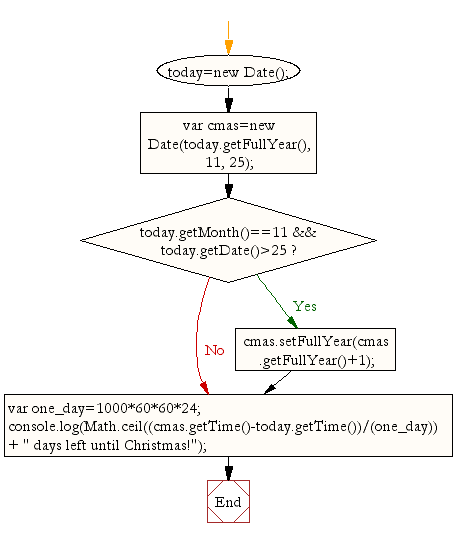# JavaScript: Calculate number of days left until next Christmas

## JavaScript Basic: Exercise-9 with Solution

Write a JavaScript program to calculate number of days left until next Christmas.

Sample Solution:

HTML Code:

``````<!DOCTYPE html>
<html>
<meta charset=utf-8 />
<title>Write a JavaScript program to calculate days left until next Christmas</title>
<body>
</body>
</html>
```
```

JavaScript Code:

``````today=new Date();
var cmas=new Date(today.getFullYear(), 11, 25);
if (today.getMonth()==11 && today.getDate()>25)
{
cmas.setFullYear(cmas.getFullYear()+1);
}
var one_day=1000*60*60*24;
console.log(Math.ceil((cmas.getTime()-today.getTime())/(one_day))+
" days left until Christmas!");
```
```

Sample Output:

```349 days left until Christmas!
```

Explanation:

Declaring a JavaScript date : In JavaScript Date objects are based on a time value that is the number of milliseconds since 1 January, 1970 UTC. You can declare a date in the following ways :

```new Date();
new Date(value);
new Date(dateString);
new Date(year, month[, day[, hour[, minutes[, seconds[, milliseconds]]]]]);
```

The getFullYear() method is used to get the year of the specified date according to local time. The value returned by the method is an absolute number. For dates between the years 1000 and 9999, getFullYear() returns a four-digit number, for example, 1985.

The getMonth() method is used to get the month in the specified date according to local time, as a zero-based value. The value returned by getMonth() is an integer between 0 and 11. 0 corresponds to January, 1 to February, and so on.

The getDate() method is used to get the day of the month for the specified date according to local time. The value returned by getDate() is an integer between 1 and 31.

The getTime() method is used to get the numeric value corresponding to the time for the specified date according to universal time.

The Math.ceil() function is used to get the smallest integer greater than or equal to a given number.

Flowchart:ES6 Version:

``````today=new Date();
const cmas=new Date(today.getFullYear(), 11, 25);
if (today.getMonth()==11 && today.getDate()>25)
{
cmas.setFullYear(cmas.getFullYear()+1);
}
const one_day=1000*60*60*24;
console.log(`\${Math.ceil((cmas.getTime()-today.getTime())/(one_day))} days left until Christmas!`);
``````

Live Demo:

See the Pen JavaScript: days left until next Christmas: basic-ex-9 by w3resource (@w3resource) on CodePen.

Improve this sample solution and post your code through Disqus

What is the difficulty level of this exercise?

Test your Programming skills with w3resource's quiz.

﻿

## JavaScript: Tips of the Day

Returns the sum of the powers of all the numbers from start to end (both inclusive)

Example:

```const sumPower = (end, power = 2, start = 1) =>
Array(end + 1 - start)
.fill(0)
.map((x, i) => (i + start) ** power)
.reduce((a, b) => a + b, 0);
console.log(sumPower(10)); // 385
console.log(sumPower(10, 3)); // 3025
console.log(sumPower(10, 3, 5)); // 2925
```

Output:

```385
3025
2925
```